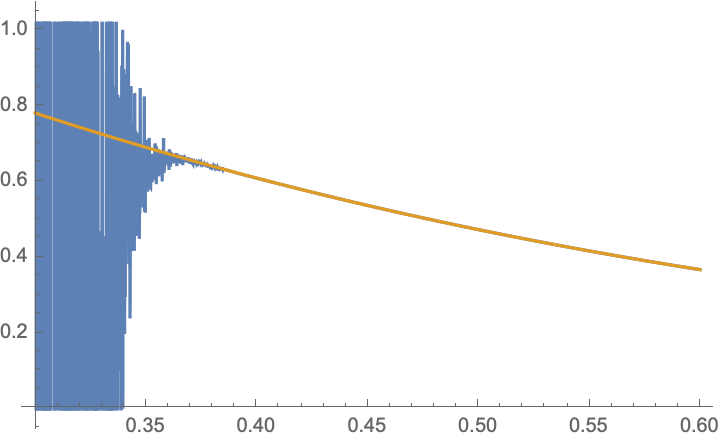# Inaccurate results for the analytical expression of $\mathbb{E}\left[ a \mathcal{Q} \left( \sqrt{b } \gamma \right) \right]$

I'm trying to plot a graph for the following expectation

$$\mathbb{E}\left[ a \mathcal{Q} \left( \sqrt{b } \gamma \right) \right]=a 2^{-\frac{\kappa }{2}-1} b^{-\frac{\kappa }{2}} \theta ^{-\kappa } \left(\frac{\, _2F_2\left(\frac{\kappa }{2}+\frac{1}{2},\frac{\kappa }{2};\frac{1}{2},\frac{\kappa }{2}+1;\frac{1}{2 b \theta ^2}\right)}{\Gamma \left(\frac{\kappa }{2}+1\right)}-\frac{\kappa \, _2F_2\left(\frac{\kappa }{2}+\frac{1}{2},\frac{\kappa }{2}+1;\frac{3}{2},\frac{\kappa }{2}+\frac{3}{2};\frac{1}{2 b \theta ^2}\right)}{\sqrt{2} \sqrt{b} \theta \Gamma \left(\frac{\kappa +3}{2}\right)}\right)$$ where $$a$$ and $$b$$ are constant values, $$\mathcal{Q}$$ is the Gaussian Q-function, which is defined as $$\mathcal{Q}(x) = \frac{1}{\sqrt{2 \pi}}\int_{x}^{\infty} e^{-u^2/2}du$$ and $$\gamma$$ is a random variable with Gamma distribition, i.e., $$f_{\gamma}(y) \sim \frac{1}{\Gamma(\kappa)\theta^{\kappa}} y^{\kappa-1} e^{-y/\theta}$$ with $$\kappa > 0$$ and $$\theta > 0$$.

This equation was also found with Mathematica, so it seems to be correct.

Follows some examples, where I have checked the analytical results against the simulated ones.

When $$\kappa = 12.85$$, $$\theta = 0.533397$$, $$a=3$$ and $$b = 1/5$$ it returns the correct value $$0.0218116$$.

When $$\kappa = 12.85$$, $$\theta = 0.475391$$, $$a=3$$ and $$b = 1/5$$ it returns the correct value $$0.0408816$$.

When $$\kappa = 12.85$$, $$\theta = 0.423692$$, $$a=3$$ and $$b = 1/5$$ it returns the value $$-1.49831$$, which is negative. However, the correct result should be a value around $$0.0585$$.

When $$\kappa = 12.85$$, $$\theta = 0.336551$$, $$a=3$$ and $$b = 1/5$$ it returns the value $$630902$$. However, the correct result should be a value around $$0.1277$$.

Therefore, the issue happens as $$\theta$$ decreases. For values of $$\theta > 0.423692$$ the analytical matches the simulated results. The issue only happens when $$\theta <= 0.423692$$.

I'd like to know if that is an accuracy issue or if I'm missing something here and if there is a way to correctly plot a graph that matches the simulation.

• This might be a bug in Mathematica kernel (I have already found one such issue with the $_2F_1$ function — Wolfram corrected it within a few months after I contacted them), but I suspect simple rounding error here: you seem to be subtracting huge values here, the hypergeometric function is of the order $10^11$ to $10^16$ for the given problematic parameters. Jul 28 '20 at 7:33
• Maybe using a higher PrecisionGoal value helps. But this might also increase the calculation time considerably. Jul 28 '20 at 8:50
• @MateuszKwaśnicki, I have also seen the same problem plotting with matlab... perhaps there is a way to simplify or derive the above expression another way with other functions.... Jul 28 '20 at 12:20
• @FelipeAugustodeFigueiredo: This is perhaps better suited to mathematica.se then. Anyway, have you tried working with higher precision? This can be done by writing SetPrecision[12.85, 50] etc. for all numeric constants. Jul 28 '20 at 12:40
• @MateuszKwaśnicki, thanks for your suggestion, with that I was able to correctly get the expected results. Jul 28 '20 at 14:59

You just need to use a higher precision, using exact numbers when possible, as shown in the Mathematica work below (with $$k:=\kappa$$ and $$t:=\theta$$). (However, this question is indeed better suited for Mathematica SE.)The accuracy issue with the evaluation of the hypergeometric function can be avoided for integer $$\kappa$$, since then the full expression reduces to an error function (see my answer to your previous question).
I tried this out for $$\kappa=5$$. Then $$\mathbb{E}\left[ a \mathcal{Q} \left( \sqrt{b } \gamma \right) \right]=\frac{a}{48 b^4 {\theta}^8} \left[\sqrt{\frac{2b}{\pi }} {\theta} \left(b {\theta}^2 \left(4 b {\theta}^2 \left(3 b {\theta}^2 \left(\sqrt{2 \pi b} {\theta}-2\right)+1\right)+1\right)+1\right)-e^{\frac{1}{2 b {\theta}^2}} \left(b {\theta}^2 \left(3 b {\theta}^2 \left(8 b^2 {\theta}^4-4 b {\theta}^2+1\right)+2\right)+1\right) \text{erfc}\left(\frac{1}{\sqrt{2b} {\theta}}\right)\right].$$
In the plot, for $$a=3,b=1/5$$ as a function of $$\theta$$, I compare the above expression with the error function (gold) with a numerical evaluation of the original expression with the hypergeometric function (blue). You see that the two evaluations agree for sufficiently large $$\theta$$, but then upon reducing $$\theta$$ the latter becomes numerically unstable while the former does not.